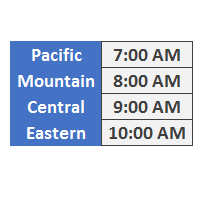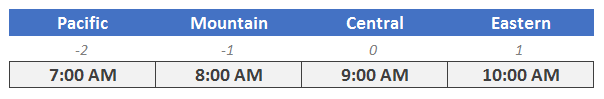I was recently working on a workbook, and had to convert an event time from one time zone to another. Basically, I wanted to enter the time of day into a cell, and then I needed a formula to compute the event time in a different time zone. This post walks through the formula to add and subtract hours, which can be helpful when translating event times between time zones.

## Objective

Before we get into the formula, let’s talk about our goal. The goal is to have an input cell that we can use to enter a time of day, such as 9:00 AM. Let’s say the entered time represents the event’s start time in the Central time zone. This is illustrated in the screenshot below.Then, under the input cell, we’d like to have formulas that compute the event start time in four US time zones. This essentially boils down to adding and subtracting hours, as shown below.Alright, let’s get to it.

## Details

It feels intuitive to simply take the event start time, for example 9:00 AM, and just add or subtract the desired number of hours. But, when you do, it doesn’t seem to have any impact. For example, if the input cell C5 had a value of 9:00 AM, and you write a formula that adds one, something like =C5+1, the result (when formatted as a time) seems to return 9:00 AM.

Excel doesn’t appear to add anything. This is because of the way Excel stores dates and times. The date part is stored to the left of the decimal, and the time part is stored to the right of the decimal. This means that adding 1 is actually adding 1 day, not one hour. If the formula cell is formatted as a time, it doesn’t display the date part, so, the formula doesn’t appear to do anything.

So far so good? Good. With this in mind, let’s turn back to our objective. We can add an hour by adding the corresponding decimal value. We know there are 24 hours in one day, so, we can get the corresponding decimal value by dividing the number of hours by 24. That means, instead of adding 1, we actually add 1/24. To add two hours, it would be 2/24, and so on.

To make the formula easier to manage, I stored the number of hours to add or subtract in row 11. Then, I essentially added (or subtracted) that number divided by 24 to the input cell C5. This is illustrated in the screenshot below.And, now you know the trick to adding and subtracting hours in Excel!

If you have any additional tips for working with times, please share by posting a comment below.

## Resources

Get a quick email notice when a new Excel article is available

• This field is for validation purposes and should be left unchanged.1.Pat Dougherty

When I change the Central time to 12:00:00am I got a string of #’s for the Pacific & Mountain time zones. I guess Excel hasn’t invented a time travel function. You need to refine this formula.

1.jefflenning Post author

Pat…ah yes, I do confirm that happens when the time value goes negative. Thanks for pointing that out! One way to handle that is to add 1 to the result. For example, instead of the formula =(\$C\$5+(B11/24)), we would update it to =(\$C\$5+(B11/24))+1. I’ve updated the workbook accordingly…and thanks again!
Thanks
Jeff

2.Barb Giss

I also get the same string of #’s for Pacific & Mountain time zones when 12:00:00 AM is the input. Expanding the columns does not change the result. When 01:00:00 AM is the input, only the Pacific time zone has the error. If you include the date component of the date / time it will work fine as long as it is after 1/0/1900.

1.jefflenning Post author

Barb … yes, thanks! I’ve uploaded a revised Excel workbook that includes two worksheets now. One for when the input cell contains both the date and time, and one worksheet for when you are using times only.
Thanks,
Jeff

3.AlexJ

Wouldn’t it be easier to use =\$C\$5+TIME(1,0,0) to add an hour? Is there a drawback to this method that I’m missing?

1.Jeff Lenning Post author
4.John Avellani